# Aligning the system from scratch¶

## Overall optical alignment¶

The fundamental positions of all optical components in the system (except the WFS head itself) should already be set. This was work that was done by (most likely) Daren Dillon, and involves making sure that the optics are all at the correct position. Of particular concern is the z-axis position, i.e. that Wfr and Twt are at conjugate pupil planes. This should have been set, validated and left.

Note

It is worth noting that the optical design is such that the actual pupil plane is not perpendicular to the optical axis in the AO relay. Talk to Brian Bauman about why this is the case.

Another very infrequent alignment is the x-y position of Wfr relative to Twt. It is important to have the two pupils well-registered in terms x-y translation between the actuator grids, rotation, and to verify the magnification (which is designed to be 5.5 Wfr actuators per Twt actuator.) This is normally tested by using the procedure that locates all the actuators relative to the lenslets.

Also of concern is the pointing through the system. There are several places where the pointing can be picked up, such as with PNC and TT. These should be aligned such that the adjustable devices operate as close to middle of range as possible. Some times things will drift, and alignments will compensate for one another. You may have to intermittently re-zero the commands on PNC and TT.

## WFS internal optics¶

This will hopefully not have to be touched, as the WFS unit itself is very small and changing and testing it is a laborious process. The most important things to get right here are the position of the doublets to obtain proper spot size and spot spacing at the image plane, proper z-axis positioning of the CCD to get best in-focus spots, and proper x/y/rotation positioning of the CCD so that the spot grid is dead on with the CCD pixel grid. For details on this, see Don Gavel’s report. “Title here.”

Reference slopes are used to pick up for non-common-path errors. We initially used just a single measurement (taken in January 2012) for the references. As time has gone on, it is clear that drifts are causing what appears to be rotation of the CCD pixels relative to the spot grid. This has necessitated adding in a rotation term (see REF_OFFS).

As of June 21, 2012, we are now able to present a bright enough light source through the pinholed spatial filter to take fresh references. This is done with IDL wrapping of the AOC’s functionality for this (see Reference Centroids). Initial results showed that the WFS amplifiers (a 8 x 2 grid across the chip) have shifted, as well as low-order modes.

## WFS positioning¶

The exact positioning of the WFS pupil (the lenslets) in the optical path is essential. We spent a large amount of time puzzling over getting this positioning right. Doing this from first principles is non-trivial. First off, there are the issues with the positioning of PNC and getting both the pupil location and beam collimation correct, while maintaining proper overall focus and SF position. Second, the tolerances of which this has to be positioned (on the order of 50 microns) are very small – the WFS head is not on an automated stage, so this is done by hand with shims.

Throughout the process we had been relying on two completely different measurements to verify position. The first one (intensity) is now the method of choice and is described in detail below. The second one (parallax) is discredited, and why that is the case is explained below as well.

The first measurement is of intensity variations in the pupil caused by phase variations on Twt. Given the Talbot lengths involved, if the WFS is not at the pupil plane, sine phase ripples on Twt will turn partly into amplitude ripples. Our standard measurement for this is by placing Fourier modes 12,12 on Twt and analyzing the intensity per subap as seen by the WFS. (If we are significantly mis-positioned this can also be seen by eye with dispraw.) The WFS head is positioned in z in an iterative process while we measure the intensity. Daren has done a very good job of getting it really close just doing this by eye, and then the final tweaks are made. We do not have a reliable quantitative model for z-position change given a certain amount of intensity fluctuation – this is done by hand.

At some points we have also used the Flea camera to directly image the intensity near the pupil plane location. These images make clear two important things. First, there are other amplitude variations in the system, in particular at the spatial frequency of the inter-actuator ripple and its harmonics. Second, the actual pupil itself is tilted relative to the z-axis. This means that we cannot ever get the entire pupil to have uniform intensity – no matter where we position the WFS one side (left or right) will be slightly ahead of the exact conjugate, and the other side of the aperture will be slightly behind. (There is a good explanation for why this occurs – ask Brian Bauman.)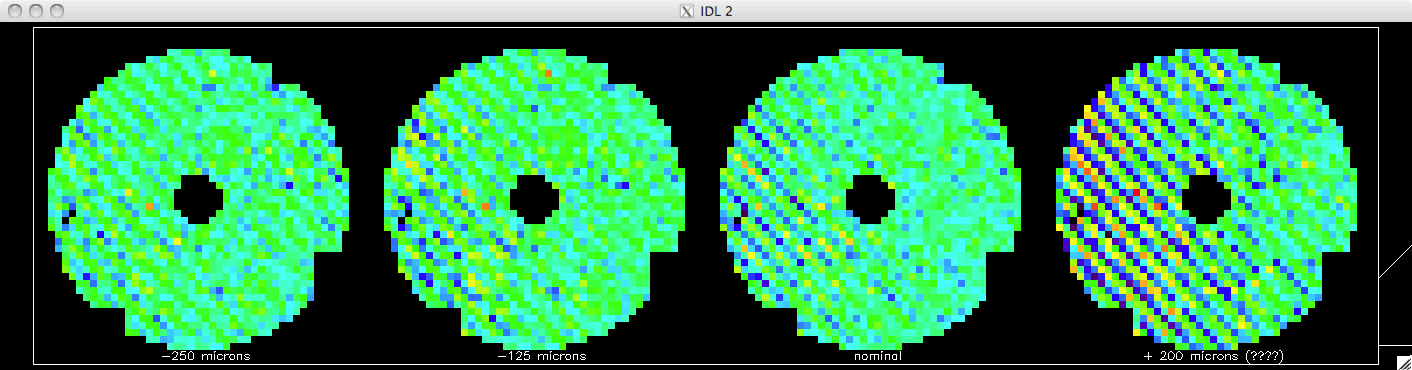Figure: Sample relative intensity measurements for Fourier mode 12,12 for the WFS positioned at different z-axis locations. We position the WFS to minimize these amplitude variations. Note the intensity pattern at +200 microns, and how it is non-uniform across the pupil along x.

The second method that we had been using to verify the pupil-conjugation of the WFS was termed parallax. If the WFS is not at the pupil plane, when the pointing is changed (either through moving TT or the ASU), the centering of the pupil will shift. This is straightforward to see with a simple drawing of the 4f relay. This was tested using code that measures the centering of Twt actuators relative to the lenslets for a variety of pointings.

The parallax and intensity methods of determining pupil conjugation of the WFS never agreed. This was quite puzzling until we went back and simulated what was happening. It turns out that with having the SF in place, even if it was opened to twice or three times nominal size, was enough to induce a centering change on poked Twt actuators when the pointing was varied, even though the simulation itself was perfectly pupil-conjugated. So we had been chasing an artifact of the measurement process, not a real misalignment. As a result the parallax test is not used and we rely on the intensity test.

Given how involved this process is, we only have to get it right once, and then bolt the WFS down. We have no data that support the z-position drifting significantly with time.

## Pixel processing of raw CCD frames¶

The raw WFS frames are processed by the AOC with both dark subtraction and flat fielding. These are specified in parms.

The flat field for the device was a complicated thing to measure (talk to Sandrine about this). We have only a single flat for the CCD and do not retake it.

The dark file is very important to dim star performance and should be taken regular. Use the IDL wrapper for AOC functionality for this (see WFS Darks).

## Unit calibration of the WFS¶

Through out the WFS build and certification process, substantial effort was devoted to verifying that the measurements of the WFS (which are initially in mas of spot motion, and can be converted to nm of spot motion through application of a known constant, and then reconstructed to nm of phase) are accurate. This involved both optical modelling (known the pixel size, the lenslet size, the wavelength, etc.) and also verifying this to known, accurate measurements. Most of the details of this should be in the WFS acceptance document written by Don Gavel.

In brief, we have tested the measurements and reconstruction of known amounts of focus (e.g. pulling back a source from the true focal point where it feeds the system) both off bench with a single OAP and on-instrument at intermediate focal points. These WFS measurements were compared to theory and to the ThorLabs WFS measurements.

Given this, we have high confidence in the WFS that it reports the correct amount of phase aberration.

## Twt actuator types¶

The MEMS Twt has several actuators that do not have a full range of voltage response. These were determined by BMC during device testing. Information on this is available in a PowerPoint file (this is on Knowledge tree at http://dms.hia.nrc.ca/view.php?fDocumentId=20125). I have taken this information and transcribed it into an IDL code load_actuator_types.

The actuators are located on the full 64 x 64 array of the Twt and then rotated and extracted to map correctly onto the specified 48 x 48 sector.

If these change, you will need to regenerate the map of valid subapertures (see WFS_CAL.fits) and the map of Twt integrator leaks (see twtLeakgains.fits).

## Misbehaving actuators: pupil selection, no special control¶

The active pupil is defined through a mask of valid suapertures. This is specified in a configuration file WFS_CAL.fits.

At the most simple this is defined based on illumination. For GPI we have used the definition of the pupil size (OD = 7.77m, ID =1.024m) and the subaperture size (d = OD/43). The default illumination criterion is that a subaperture is valid if it is at least 50% illuminated.

In practice, however, we have had to be a bit more stringent with regards to the central obscuration. Using our baseline definition, the circle of invalid subaps in the center is five across (forming a 3-5-5-5-3 circle, row by row). In practice at UCSC, we have had to enlarge this slightly so that it is 7 subaps across (a 3-5-7-7-7-5-3 circle, row by row.)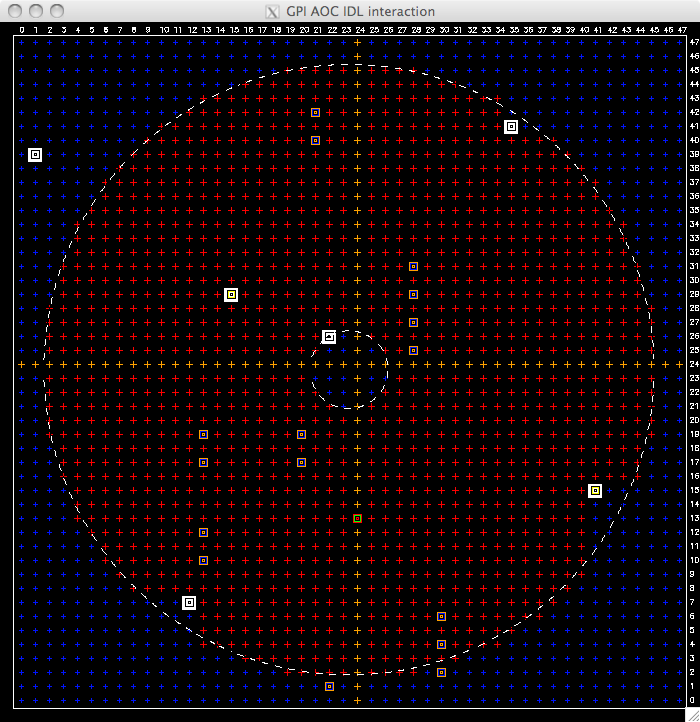Figure

We also use the subap mask to remove non-responsive actuators from the active pupil. These actuators are problematic for two reasons. We can measure their position, but since we cannot control them, we will always measure an error signal. This leads to integrator windup, saturation, clipping and cleanup, none of which help. The best solution is to simply mask off the four subapertures that immediately surround a non-responsive actuators. Per our map of the actuator types (see Twt actuator types) we use the “maskout” variable returned by load_actuator_types.pro, which for the GPI pupil is shown in the Figure above.

Though there are many other actuators that are aberrant as marked by BMC, none have required any special calibrations. Of all of the “coupled” actuators, only the coupled actuators at GPI coordinates (20,17 and 20,19) and the four at (28,25 and 28,27 and 28,29 and 28,31) have shown evidence of extra residual error during closed loop performance. This level is low enough that we have not needed to implement an explicitly coupled scheme for controlling them. (For details on the independent actuator voltage-phase control, see Slaving of actuators outside the pupil).

For dead actuators near the edge of the pupil (which all of ours are), simply masking just the 2 x 2 box around does not necessarily result in best performance. For example, if we just zero-out the 2x2 subaps around the dead actuators on the GPI default pupil, we get the valid mask as shown on the left side of the Figure below. The small one-subap across regions right near the edge lead to artifacts during reconstruction. For most robust performance and stability we have settled upon blocking off the dead actuators as if sticking in a square corner from outside to block off the subapertures. See right side of the figure. This should be (but remains to be verified) final for final science image performance, as these sections of the pupil are blocked by the Lyot plane mask.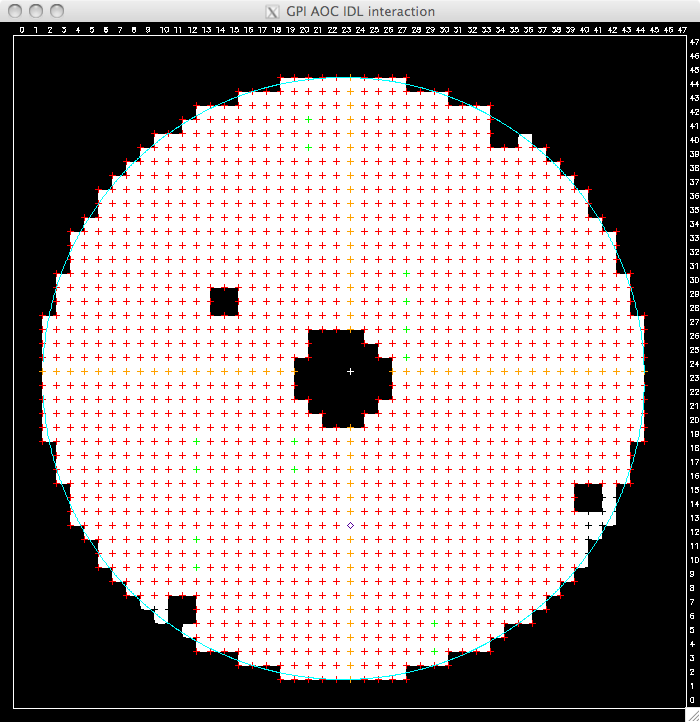Figure

Valid subapmask with two x two regions around dead actuators blocked off. Note the one-subap across regions near the edge.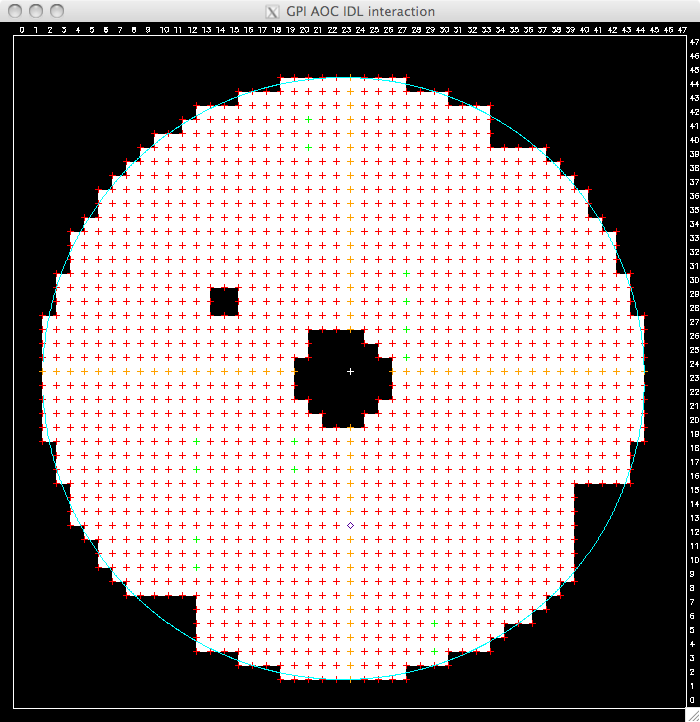Figure: with those one-subap excursions removed, which is effectively like sticking a square corner into the pupil from outside to mask off the dead actuator.

Use code

IDL> ult_make_subapmask


To regenerate this given the current dead actuator mapping.

## Reconstruction filter¶

The FTR algorithm requires a filter. The filter describes the behavior of the WFS. In GPI, we separate out the WFS from the DMs. First we use FTR to estimate the phase, not the Dm commands. Second, we use a combination of the MTA and the influence function filter for the Twt to determine the best commands. As such, our reconstruction filter should describe GPI’s WFS as well as possible.

In general, we have three options for creating a filter. First, we could use a purely theoretical filter based on a model. Second, we could use a computer simulation to calculate the filter for GPI’s nominal design, Third, we could try to meaure a ‘poke’ filter, in essence by putting Fourier mode on the system and analyzing WFS measurements.

Method three does not work well in practice, due to both noise and the entanglement of the Twt influence function. We have code that produces filters for the other two options.

Our default filter is method 2: it is based on the detailed end-to-end GPI AO simulator. This should capture the behavior of the Quadcell Shack-Hartmann. Since this was measured in the simulator, the file itself is saved in the IDL distribution. If it ever has to be recreated it will require Lisa running local code. To instantiate it into parms.txt on the aoc, run

IDL> ult_make_ftr_filter


In case it is ever needed for testing, we also include the first option, a purely theoretical filter.

IDL> ult_make_ftr_filter, /modhud


Uses the “modified hudgin” geometry filter (see Eq. 15 and 16 of Poyneer, JOSA A 2005). As compared to the formula this enforces Hermitian symmetry across the full domain and is conjugated and transposed to be saved in the file format specified for the AOC.

Note: If the conjugation is wrong, this reconstruction results in a phase with has the wrong sign (+ vs. -) and is shifted by one whole actuator in each direction. We actually used the system for a while in 2009 in this mistaken configuration – weird things will start to happen if you have this. You are forewarned.

## Deconstruction filter¶

The FTR filter (above) goes from slopes to phase. We also need to go from phase to slopes. Internal to the AOC this is done to the phase measurements from the Cal system. The file is specified as exactly the same full FTR filter as above; the AOC (and the IDL code) just applies it forward not inverse. By default we use the same FTR filter as for recon. To reset this to the default value, use

IDL> ult_make_cal_filter  ;; also takes /modhud flag if so desired


However, due to various considerations with the Cal system, we may want to restrict this to just the LOWFS. To do so call

IDL> ult_make_cal_filter, /lowfs


Which will restrict the frequencies to only those that the LOWFS can easily see and report (otherwise the ref offsets will wind up due to unobserved modes.)

Note that the IDL code right now (specifically rtc_do_refs_from_phase.pro) uses the TWT_FTR_FLTR file to make refcents, as we use this for speckle nulling and the like.

## Wfr-Twt simultaneous control¶

We presently use Wfr and Twt simultaneously and split the spatial frequency content of the phase correction between them. This is an algorithm that was developed during GPI design (see Lavigne JOSA A 2008). The fundamental concept is that a specific set of low-order Fourier modes are selected to go on Wfr. These coefficients are extracted out of the reconstructed phase. The real and imaginary parts of the coefficients are put into a vector, which is multiplied by a matrix. This matrix, the Modes-to-actuators matrix (MTA) is specified in parms.

The MTA is measured directly on the system, which means that it captures the relative positions of the Wfr actuators to the Twt actuator grid, and also captures the influence function response of Wfr. To measure this in IDL do as follows.

Locate the actuators. First do this for the Twt

IDL> ult_locate_actuators, /tweet


Then do it for the Wfr

IDL> ult_locate_actuators, /woof


The alignment between the Wfr and Twt is set once (see Overall optical alignment). The alignment of the Twt to the lenslets has to be tweaked regularly (see System Alignment). The codes will save the locations to disk, which can be viewed graphically with

IDL> new_fancy_display_locations


and will print out information on the alignment. From my MTA measurement on June 14, 2012: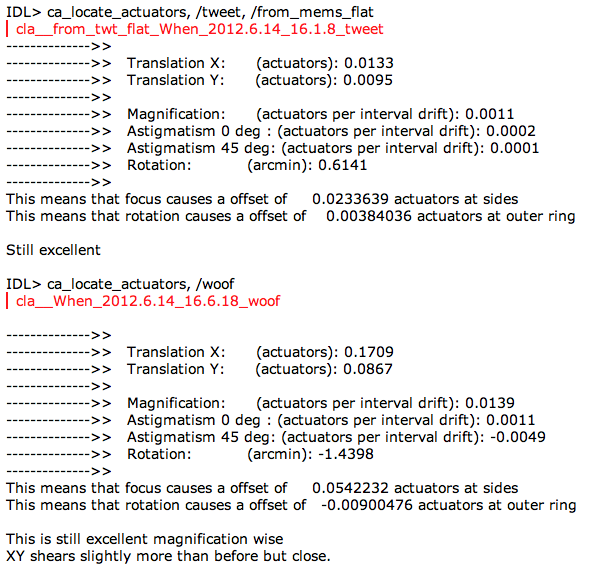Figure: Sample results from actuator location measurement during MTA generation

Now that the exact locations are known, the next step is to measure the influence functions and produce the matrix. This is done by

IDL> ult_make_mta


This code does as follows. First, poke each actuator and measure the influence function. It then aligns them (based on actuator locations) and smooths them to produce a composite best-fit influence function measurement. Then a data cube consisting of the estimate of what each actuator poke looks like is generated for all actuators on the Wfr . (This processes is done for two reasons. First, measurements will have noise, and we want to clean that up so it does not get inverted. Second, many Wfr actuators are near the edge or outside of the Twt pupil. These will be measured only partly by the actual WFS, but we need the full response for the MTA. Our process allows us to estimate that.)

Next the code prompts you to enter the spatial frequency range that will be handed off to Wfr. There is a default value tat was set by our design study to maximize the power offloaded while preventing errors and partial mode correction (which would necessitate feedback; see Lavigne’s paper).

Now the code calculates a forward matrix. This uses the best-estimate Wfr pokes to generate matrix that has as each column the phase that is measured by the WFS-FTR for a given Wfr actuator. This is a Wfr actuator poke to phase matrix. This matrix is inverted with SVD to produce a reverse matrix, which goes from phase points in the Twt grid to Wfr actuators.

The next step is to construct a so-called desired matrix. This has as each column the matrix-vector multiplication of the reverse matrix with the phase on the Twt grid produced by each of the sine or cosine Fourier modes that the will be sent to the Wfr. In other words, this is the matrix of coefficients of Fourier modes of the Wfr to Wfr actuators. This matrix is our ftcos_to_woofer matrix.

To ensure that no piston goes on the mirror, a simple piston removal matrix is generated and multiplied in front to ftcos_to_woofer. The matrix for the reverse process (Wfr actuators to coefficients of Fourier modes on the Wfr) is generated with SVD.

The code stops and asks you to examine. If the matrix is acceptable, all files are saved in the locations pointed to by parms. They are also archived in private with a time stamp for potential later analysis.

Once the MTA has been created, we need to verify that it has unity gain for all the modes. This is done with

IDL> ult_tweak_mta


This code places all the low-order Fourier modes on Wfr via MTA, and compares the RMS value of what it measures with the desired amount. This commanded-measured gain should be one for all modes. The Figure below shows an example of two modes tested with this code. It usually works out to be very close to 1, and so further tweaking of the matrix is necessary. If there is an overall non-unity gain, that probably means that something else has changed and been mis-calibrated, e.g. the Wfr phase-voltage curve was changed or the light source has the wrong spectrum, significantly changing the spot size.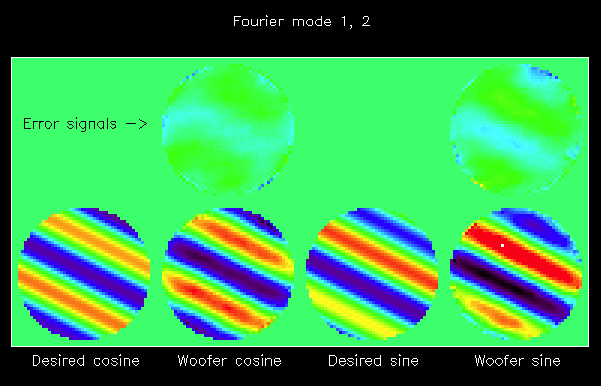Figure

Desired low-order Fourier modes and the measured Fourier modes, as placed on Wfr after generation of a new MTA. (code: ca_tweak.pro)

There are a couple of quirks of the Fourier-domain modal split that should be respected.

First, the split is done on the entire phase signal in the square grid, not just the actuators. The method of slope edge correction that is used is designed to estimate the phase as well as possible inside the aperture, and make the phase as flat (and = 0) as possible outside the aperture. One outcome of this is that if the full phase has a large excursion near the edge, it will introduce a sharp discontinuity at the edge of the Twt commands. Only three modes that we encounter really have this problem – focus and both astigmatisms. Focus in particular is a problem in GPI as it is currently set up. There is a large amount of focus in the AO relay. This can (of course) be easily picked up by Wfr. However, when the large focus is seen and split by AO, what results is a nice focus shape in Wfr, but the Twt has a sharp discontinuity at the edge. This excursion, which is a few hundred nm in amplitude, is an artifact and can lead to stability problems with the SF closed. Therefore we use an offset file (see DM Flats) to add the focus on the Wfr automatically.

## Twt influence function precompensation filter¶

The Twt has a large variation in amplification of signals placed on it as a function of spatial frequency. We have known this for quite some time, and have an established method to pre-compensate for this.

The theory behind this filter is described in this paper (downloadable PDF file).

Low spatial frequencies on the MEMS are amplified, while high spatial frequencies are attenuated. For the same rms input Fourier mode on the Twt (in phase), the resulting actual phase on the mirror varies by a factor of ten (rms) from low to high spatial frequencies.

To produce the best single-step phase shaping, and to get best closed-loop bandwidth, we must use a precompensation filter. This filter is essentially the Fourier transform of a single influence function, appropriately sampled. This filter is applied with division to the phase in the Fourier domain.

There are at least three ways to define this influence function filter. First, we can use a high-resolution measurement of the influence function (which is usually obtained with the PSDI). Using the appropriate padding, this can be Fourier transformed to directly obtain the transfer function of the mirror. This is then fit with a model. This will capture the behavior of Twt only.

A second option is to measure the modal gains in situ with the AO system. This involves placing a selection of Fourier modes on Twt and then measuring them. The effective command-measurement gain is calculated via RMS power. This has the advantage that it captures everything in the system. Two factors in particular may be important. This captures any difference in behavior of the actual WFS measurement process from the discretized model that we use (see Reconstruction filter). It also can capture effects of the SF, such as measuring lower amplitude for modes past a certain spatial frequency, due to the higher order diffraction terms (harmonics) being cut off by the SF. On the other hand, this type of measurement is subject to a noise and artifacts. To ameliorate this, the command-measurement gains are fit with a model.

A final way to set this is just to guess at something.

Once you have a new filter, you need to store it at TWT_INF_FUNC_MASK and then select it for use. Though you can read in TWT_INF_FUNC_MASK, there is no data dump to tell what is actually being used (e.g. ones or host). To verify, you have to analyze long time series of telemetry with IDL helpers. To diagnose this, use the finaltests/test_cl_psd3_tt_ho.pro or finaltests/test_cl_psd4_ofc.pro codes to estimate the modal gains in closed loop. This works OK with just the light source, but it can be done nicely on a weak phase plate as well. An example of this testing, using either all ones for the filter or the specified filter, is shown below.

On the left is the case where no influence function compensation was used. Since the MTA guarantees unity gain for Wfr modes (see Deconstruction filter). They appear sharply different. The large amplification of low spatial frequencies that Twt has is clear in comparison. The amplification lessens as spatial frequency increases. For the highest spatial frequency modes the gain is less than unity (dark purple to black) in the figure. On the right is the system gain map when influence function compensation is used. This is much more uniform, across nearly all the spatial frequency range. The orange-colored higher gains at top and bottom are most likely due to a misalignment of the SF pointing (without the SF we see much higher gains at top, bottom, left and right). The gains are still low at the very highest spatial frequencies because of noise-suppression for local waffle.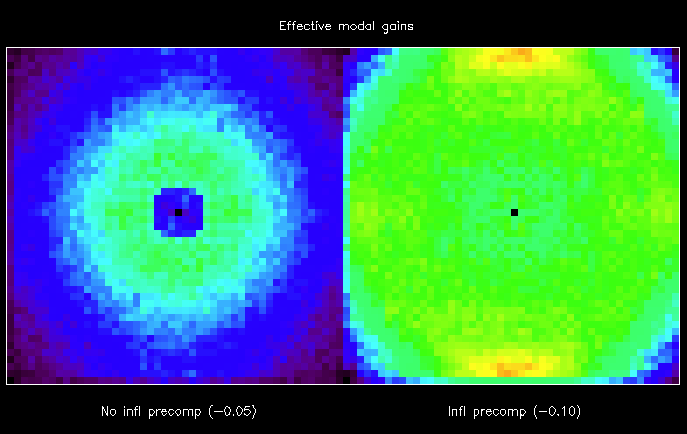Figure: Estimated closed-loop Fourier modal gains. In this case the left panel shows the gains when no influence function precompensation is used. On the right is a well-calibrated TWT_INF_FUNC_MASK. Note that the Wfr modes are clearly visible on the left. This is because the are extracted before the TWT_INF_FUNC_MASK is applied, so it does not affect their gains.

## Tricks you can play with the infl filter¶

As mentioned above, the filter can compensate not just for the response of Twt, but other factors in the system.

Our default goal is to have unity-gains on all modes. As a practical matter, that has not turned out to be always desirable. In particular the AOC system is susceptible to local waffle. The AOC has built-in functionality to remove this from Twt commands (see ?? ). However, we can also alleviate this problem by lowering the effective modal gain for those modes.

The influence function filter is defined in a forward sense – it is the amplification or attenuation of a command placed on Twt. It is applied internally through division. So to lower the gain of the modes near waffle, the filter that is used has larger numbers near waffle. This is usually accomplished by adding or multiplying a Gaussian of a few pixels standard dev around waffle.

Another concern is just how much Twt attenuates the higher frequency modes. We can place a hard limit on the pre-amplification of these modes either by directly manipulating the filter itself before we save it, or with KEYWORD.

## Wfr phase integrator value¶

The Wfr and Twt residual phases are integrated separately after they are split. The integrations are done in the spatial (actuator) domain. The Wfr Dm integrator value is set in parms (see CENTROID_X_GAIN).

A higher integrator memory (e.g. 0.999) leads to better rejections of very slowly varying (or static) errors, but it takes longer from problems to ‘leak off’ the DMs. Setting this is a tradeoff between rejection and stability.

Presently, the best value for the Wfr integrator memory is 0.998, which provides (both with theory and from my experiments) 100x rejection. E.g. 100 nm RMS static focus in the system is corrected at the 99 nm level, leaving 1 nm RMS focus in the steady-state residual. (This is equivalent to a ETF with 10^4 power rejection at DC). (The previous value had been 0.99, which gives 20x rejection, or 5 nm RMS focus left over)

## Twt phase integrator - per actuator leaks¶

During initial AO integration at test at UCSC (see May 2011 results) we had stability problems with the SF closed down to desired size, when light levels were low or phase aberrations were very strong. Instabilites were generated from a specific subset of actuators, those that were either poorly controlled (right on the edge) or neighboring dead actuators. At the time, the best way to fix this was to slave these actuators (see Slaving of actuators outside the pupil).

A set of detailed Fourier optics simulations of the SFWFS were conducted by Lisa in Aug/Sep 2011. These indicated that the instabilities arose due to non-linearities through the SFWFS. For these dead neighbors and edge actuators (which border a single partially-illuminated subaperture), wind up would occur. Even a single 1 nm bias on the slope estimate, if a constant bias due to mis-positioned dead actuator or partial illumination, would accumulate on the integrator and drive an actuator far out of position, eventually losing all light in the that subaperture and driving the entire system unstable.

However, these detailed simulations also indicated that the best software solution would be to selectively lower the integrator gain (aka memory value) for actuators immediately next to the dead actuators or that were poorly controlled. This caused the bias phase to leak off instead of winding up, greatly enhancing stability.

There is code that generates and stores the best individual Twt actuator integrator memory values

IDL> ult_twt_leak_map


In this method all actuators in the 48 x 48 grid begin with an integrator memory of 0.99. Selective actuators are then set to 0.9. These actuators are: any actuator that is a dead actuator, any actuator that is immediately adjacent to a known dead actuator; any actuator the borders only one valid subaperture.

Testing results indicate that this is the most stable configuration.

## Voltage-phase (or is the voltage-surface) calibrations for all mirrors¶

When constructing the Twt voltage-phase file, there are some actuators that must be dealt with in a special fashion.

There are five actuators that are dead within the 48 x 48 portiono f the Twt that the AOC controls. Per BMC, these must always be set to 0 V. To make this happen, the fourth and fifth entries in the calibration, which set the minimum and maximum voltage that can be commanded, are both set to 0V. In practice, this means that no matter what phase or volts is commanded to the MEMS, in open or closed loop, those actuators are always clipped to 0V (For more, see the clipping/cleanup discussion in Modal cleanup). In closed-loop operating the voltages are clipped, and this information is used to update the integrator memory. The phase values of these five dead actuators should be 0 microns, always. To ensure this, we need to have the coefficients of the quadratic (specified in the first through third entries for each actuator) set such that the curve produces 0 phase at 0 V. This simply means the first entry = 0)

Another actuator that has a special voltage-phase calibration is troublesome actuator 15,29. As of Dec 2012, this is set to finish.

## Wfr offset file¶

The Wfr offset file is used to store a pure focus offset. The generation of this file has been automated - see DM Flats for details.

## TT: LQG¶

The TT LQG filter is a special filter designed to reject vibrations in the system. The theory of how this works is found in this paper (downloadable PDF file). The only significant change from the theory presented in that paper is that we are using a simple first-order AR(1) model of the atmosphere. We had stability issues using higher order models.

We also are using a fractional delay, which the system model (as opposed to the vibration model) takes care of. See this paper (downloadable PDF file). for the details.

In brief, the TT LQG filter works as follows.

We construct a state space model of the AO control system and the input aberrations. We use a state vector $${\bf x}[t]$$, which is defined as

${\bf x}[t] = ( {\bf a}[t], \phi[t-1] , {\bf b}[t], \rho[t-1])^T$

The vector $${\bf a}[t]$$ contains the states for the atmospheric TT model and for the common-path vibrations. The variable $$\phi[t-1]$$ is the total common-path phase aberration at the previous time step.

The vector $${\bf b}[t]$$ contains the states for the non-common-path vibrations. The variable $$\rho[t-1]$$ is the total non-common-path phase aberration at the previous time step.

The AOC is hardcoded with this model, as are the IDL helpers which create the right files. As of now we have three states for the atmosphere, two states each for five common-path vibrations, and two states each for five non-common-path vibrations.

The state update equation captures the evolution of the atmosphere and vibrations with each time step.

${\bf x}[t+1] = {\bf A x}[t] + {\bf B w}[t]$

The matrix $${\bf A}$$ contains the parameters which describe the models for the atmosphere and the two types of vibrations. These values change depending on the conditions.

The atmosphere is given as an AR(1) process. In our paper we had presented higher order models, but in practice with GPI we are not using them. This is because we are having stability issues when using them, and performance with the AR(1) model seems sufficient.

The exact values for $${\bf A}$$ are calculated for you based on the specifications that you give in the file is ultimate/ult_make_lqg.pro.

In the function glqgp_lookup_case, you specify the model as follows.

For the atmosphere, you set an integrator memory with variable integ. Like keyword LEAK_GAIN_TT, this is a number close to but less than 1.

For each vibration, you give the temporal frequency of the vibration in Hz via the vector variable vib_hz, and set the power (which is the rms squared) in mas in the vector variable vib_power. For example, two vibrations at 60 and 83 Hz with 2 and 4 mas respectively would be given as

IDL> vib_hz = [60., 83., 0., 0., 0]
IDL> vib_power = [2., 4., 0., 0., 0.]^2


You do the same for the non-common-path vibrations.

For any unused vibration slots, leave the power levels at 0.

The final specification that you need in is the noise level. Unfortunately this is not unit calibration, so it’s qualitative rather than quantitative. Set the noise level via the variable noise to be something in the range of 100 to 10,000. In testing, we have shown that for no vibrations, the DC rejection of the LQG is very similar to that of the regular integral controller with the following noise levels and TT_GAIN_STAGE

[lisa - finish this]

The state space model continues with the measurement equations

${\bf y}[t] = {\bf C x}[t] + {\bf D u}[t] + v[t]$

In this case the matrix $${\bf C}$$ pick out the common and non-common phase seen by the WFS that is returned in the measurement at time $$t$$.

The vector $${\bf u}[t]$$ contains the DM commands (which compensate for TT) at various points in time. The matrix $${\bf D}$$ picks out the correct ones that correspond to the commands during the WFS integration. (In the case of TT, the commands are placed asynchronously on the TT stage and Wfr surface relative to the WFS framing. This is where the arbitrary delay paper mentioned above comes in.

Warning

Following the notation in that paper, the delay $$\tau = 1.25 T$$ and the value of $$\Delta$$ is 0.25.

This makes the matrix $${\bf D} = [-0.75, -0.25]$$, following the fourth case in equation 15.

In the arbitrary delay case, the controller estimates the DM command as the linear combination of the phase as seen at two different points in the future, given all the previous measurements. Following Eq. 6 of the arbitrary delay paper, we have

$d[t+1] = (1-\Delta)\hat{\phi}[t+1 | t] + \Delta\hat{\phi}[t+2 | t]$

This is obtained in the steady state through use of the estimation equation and related ones:

\begin{align}\begin{aligned}{\bf \hat{x}}[t|t] = ({\bf I} - {\bf K_s C}){\bf A} {\bf \hat{x}}[t-1|t-1] + {\bf K_s }( y[t] - {\bf D u}[t])\\{\bf K_s} = {\bf P_s C}^H ({{\bf C P_s C}^H + {\bf P_v}})^{-1}\\{\bf P_s} = {\bf A P_s A}^H + {\bf B P_w B}^H - {\bf A P_s C}^H ({\bf C P_s C}^H + {\bf P_v})^{-1} {\bf C P_s A}^H\end{aligned}\end{align}

Note that $${\bf \hat{x}}[t|t]$$ contains the phase estimate $$\phi[t-1]$$, so to get the estimates used above for the DM command, we must calculate

\begin{align}\begin{aligned}{\bf \hat{x}}[t+1|t] = {\bf A}{\bf \hat{x}}[t|t]\\{\bf \hat{x}}[t+2|t] = {\bf A}{\bf A}{\bf \hat{x}}[t|t]\\{\bf \hat{x}}[t+3|t] = {\bf A}{\bf A}{\bf A}{\bf \hat{x}}[t|t]\end{aligned}\end{align}

We then extract the correct element to get $$\phi[t+1 | t]$$ from $${\bf \hat{x}}[t+2|t]$$ and $$\phi[t+2 | t]$$ from $${\bf \hat{x}}[t+3|t]$$.

The code in ult_make_lqg calculates all of these things for you correctly.